# Test: Modem Digital Modulation & Detection Techniques

## 10 Questions MCQ Test GATE ECE (Electronics) 2023 Mock Test Series | Test: Modem Digital Modulation & Detection Techniques

Description
Attempt Test: Modem Digital Modulation & Detection Techniques | 10 questions in 30 minutes | Mock test for GATE preparation | Free important questions MCQ to study GATE ECE (Electronics) 2023 Mock Test Series for GATE Exam | Download free PDF with solutions
QUESTION: 1

### Assertion (A): Binary phase shift keying (BPSK) is the most efficient of the three digital modulation, i.e., ASK, FSK and PSK. Reason (R): In BPSK, phase of sinusoidal carrier is changed according to the data bit to be transmitted.

Solution:

BPSK is the most efficient of the three digital modulation, i.e. ASK, FSK and PSK because BPSK is used for high bit rates. Reason is also a true statement but not the correct explanation of assertion.

QUESTION: 2

Solution:
QUESTION: 3

### Match List-I with List-ll and select the correct answer using the codes given below the lists: List-I A. BPSK B. AM C. QPSK D. ASK  List-ll 1. A group of two binary bits is represented by one phase state. 2. A binary bit is represented by one phase state. 3. Carrier ON or OFF depending on whether ‘V or ‘0’ is to be transmitted. 4. Detected using envelope detector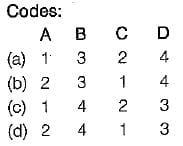Solution:
QUESTION: 4

The bit rate of a digital communication system is 34 Mbps. The modulation scheme is QPSK. The baud rate of the system is

Solution:

In BPSK, baud rate is half the bit rate due to which there is more effective utilization of the available bandwidth of the transmission channel. Given, bit rate = 34 Mbps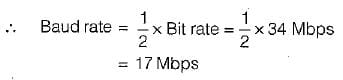QUESTION: 5

Consider the following statements;
1. BPSK has a bandwidth which is lower than that of a BFSK signal.
2. BPSK yields the maximum value of probability of error compared to ail the three digital modulation techniques i.e. ASK, FSK and PSK.
3. Binary FSK has the highest system complexity.
4. Binary ASK is demodulated using coherent detection while binary FSK and PSK are demodulated using envelope detection

Which of the statements given above are correct?

Solution:
• Statement-1 is correct.
• BPSK has the best performance of all the three digital modulation techniques in presence of noise, it yields the minimum value of probability of error compared to ASK, FSK and PSK. Hence, statement-2 is false.
• Statement-3 is correct since binary FSK has highest system complexity while binary ASK has least system complexity.
• Binary ASK is demodulated using envelope detection while binary FSK and PSK are demodulated using coherent detection.

Hence, statement-4 is fals

QUESTION: 6

Comparison of MSKand QPSK scheme shows that

Solution:

More energy (or power) is contained in the main lobe of MSK (about 99% of the total energy or power) as compared to the QPSK (about 90% of the total energy or power).

QUESTION: 7

In a digital continuous-time communication system, the bit rate of NRZ data stream is 1 Mbps and carrier frequency of transmission is 100 MHz. What is the bandwidth requirement of the channel in BPSK and QPSK systems respectively?

Solution:

The bit period of NRZ data stream is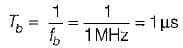In BPSK, each binary bit is a symbol, therefore symbol duration Ts = Tb = 1 μs.
∴ The bandwidth required for BPSK system is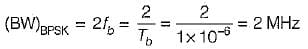In QPSK, we group two successive bits to form one symbol, therefore symbol duration,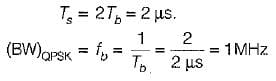QUESTION: 8

The error probability of QPSK is

Solution:

The error probability of QPSK is better (or lower) than that of BPSK due to which QPSK is used for very high bit rate data transmission.

QUESTION: 9

Bandwidth of BPSK is ___ than that of BFSK.

Solution:

Minimum bandwidth required in BPSK = 2fb.
Minimum bandwidth required in BFSK = 4fb.
Hence, bandwidth of BPSK is lower than that of BFSK.

QUESTION: 10

The error probability of QPSK is

Solution:

The error probability of QPSK is better (or lower) than that of BPSK due to which QPSK is used for very high bit rate data transmission.Use Code STAYHOME200 and get INR 200 additional OFF Use Coupon Code